### 统计语言模型

《数学之美》中是如此介绍统计语言模型的：

####P(S)=P(W1,W2,…,Wn)

####P(W1,W2,…,Wn) = P(W1)P(W2|W1)…P(Wn|W1,W2,…,Wn-1)

####log[P(S)] ####=log[P(W1)P(W2|W1)P(W3|W2)…P(Wi|Wi-1)…P(Wn|Wn-1)] ####=log[P(W1)]+log[P(W2|W1)]+log[P(W3|W2)]+…+log[P(Wi|Wi-1)]+…+log[P(Wn|Wn-1)]

### 数据准备

####P(Wi) = C(Wi)/C ####P(Wi|Wi-1) = C(Wi-1,Wi)/C(Wi-1)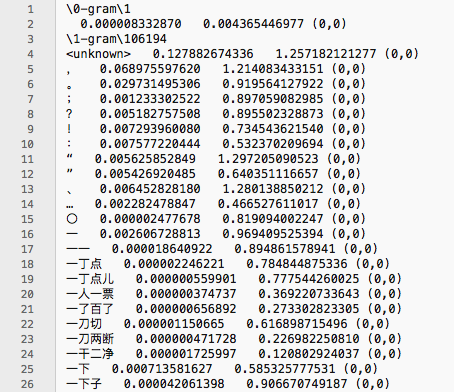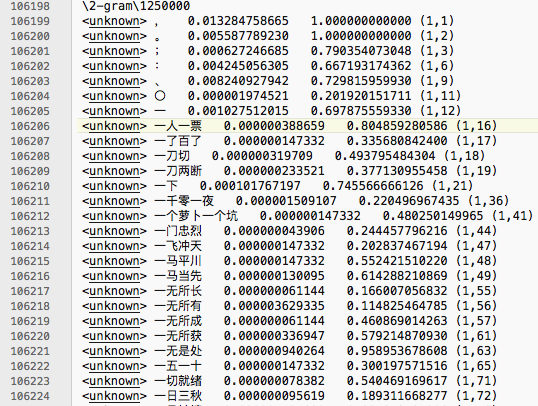static long[] byteTable = createLookupTable();
static long HSTART = 1349303770470715811L;
static long HMULT = 7664345821815920749L;

private static long[] createLookupTable()
{
long[] byteTable = new long;
long h = 0x544B2FBACAAF1684L;
for (int i = 0; i < 256; i++)
{
for (int j = 0; j < 31; j++)
{
h = (h >> 7) ^ h;
h = (h << 11) ^ h;
h = (h >> 10) ^ h;
}
byteTable[i] = h;
}
return byteTable;
}
public static long hashCode(String cs)
{
long h = HSTART;
long hmult = HMULT;
long[] ht = byteTable;
int len = cs.Length;
for (int i = 0; i < len; i++)
{
char ch = cs[i];
h = (h * hmult) ^ ht[ch & 0xff];
h = (h * hmult) ^ ht[(ch >> 8) & 0xff];
}
return h;
}


CREATE TABLE "hashtable" ("hashcode" integer ,"probability" float );


CREATE UNIQUE INDEX "hashtable_hashcode" on "hashtable"("hashcode");


### 图的定义

public class Graph
{
/***
* 顶点的定义
*/
public class Vertex
{
public List<Edge> from;//入边的集合
public List<Edge> to;//出边的集合
public object data;
public int id;

public Vertex(int id)
{
this.from = new List<Edge>();
this.to = new List<Edge>();
this.data = null;
this.id = id;
}
public override string ToString()
{
return data.ToString();
}

}
/***
* 边的定义
*/
public class Edge
{
public Vertex from;//指出该边的顶点
public Vertex to;//边所指向的顶点
public object data;

public Edge(Vertex from, Vertex to)
{
this.from = from;
this.to = to;
this.data = null;
}

}

protected Vertex[] vertex;//图中所有的顶点
protected int vertex_count = 0;//顶点的数量
protected int edge_count = 0;//边的数量

public int VertexCount
{
get { return vertex_count; }
}

public int EdgeCount
{
get { return edge_count; }
}

protected void InitializeVertex()
{
vertex = new Vertex[vertex_count];
for (int i = 0; i < vertex_count; i++)
{
vertex[i] = new Vertex(i);

}
}

/**
* 添加一条边的方法
*
*/
protected virtual Edge AddEdge(Vertex from, Vertex to)
{
Edge e = new Edge(from, to);
edge_count++;
return e;
}

/**
* 返回给定顶点的所有出边
*/
{
return Vertexes[v].to;
}

/**
* 返回给定顶点的所有出边所指向的顶点的索引；
*/
{
var result = new List<int>();
var edges = vertex[v].to;
foreach (var i in edges)
{
}
return result;
}

public int getIndex(Vertex i)
{
return i.id;
}

public Vertex[] Vertexes
{
get { return vertex; }
}

{
get { return edges; }
}
}


### 建立拼音图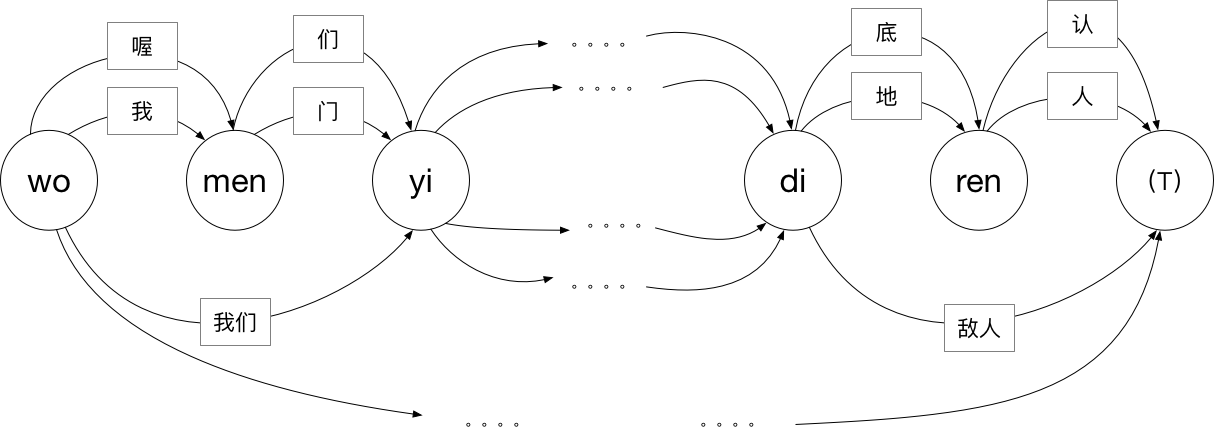public class LexiconGraph : Graph
{

public LexiconGraph()
{
}

public LexiconGraph(String sheng, String yun)
{
/*
* 顶点的数量比拼音的数量多一个，对应图中的（T），
* 如果没有这个顶点，为单字添加边时就会形成自环
*/
vertex_count = sheng.Length + 1;
InitializeVertex();

for (int i = 1; i < 5; i++)
{
for (int j = 0; i + j <= sheng.Length; j++)
{

var list = LexiconLib.Instance.getPhrases(sheng.Substring(j, i), yun.Substring(j, i));

foreach (var v in list)
{
Edge e = AddEdge(vertex[j], vertex[i + j]);
var data = new EdgeData();
data.phrase = v;
data.id = this.edge_count;
e.data = data;
}
}
}
}
/**
* 用于携带词组信息的类
*/
public class EdgeData
{
public int id;
public string phrase;
public EdgeData()
{
}
}
}


public class LexiconLib {

Dictionary<String, List<String>> zis = new Dictionary<string, List<String>>();
Dictionary<String, List<String>> cis = new Dictionary<string, List<String>>();

static LexiconLib instance;

public static LexiconLib Instance {
get {
if (instance == null) {
instance = new LexiconLib();
}
return instance;
}

}

public LexiconLib() {
}

public void clearCis() {
cis.Clear();
}

public List<String> getPhrases(String sheng, String yun) {

String key = sheng + yun;
if (sheng.Length == 1) {
if (!zis.ContainsKey(key)) {
StringBuilder stringBuilder = new StringBuilder("select distinct * from zi where ");
stringBuilder.Append("order by fre desc limit 15;");
var list = (from e in SuiHanConnetionFactory.MainDataBase.Query<Zi>(stringBuilder.ToString(), new String[] { sheng, yun }) select e.Str).ToList();
return list;
}
return zis[key];
} else {
if (!cis.ContainsKey(key)) {
StringBuilder stringBuilder = new StringBuilder("select distinct * from ci where ");
stringBuilder.Append("order by fre desc limit 10;");
var list = (from e in SuiHanConnetionFactory.MainDataBase.Query<Ci>(stringBuilder.ToString(), new String[] { sheng, yun }) select e.Str).ToList();
return list;
}
return cis[key];

}

}

static void addCondition(string sheng, string yun, StringBuilder stringBuilder) {
stringBuilder.Append(sheng.Contains("?") ? "sheng glob ? " : "sheng = ?");
stringBuilder.Append(" and ");
stringBuilder.Append(yun.Contains("?") ? "yun glob  ? " : "yun = ?");
}
}


order by fre desc limit 10;语句则是对查询结果进行排序，并限制其数量，词频太靠后的词组价值不大，我们没有必要取出所有的词组，在省略输入时尤其如此。而限制取词的数量，可以极大地提高引擎的整句速度。

### 建立词组图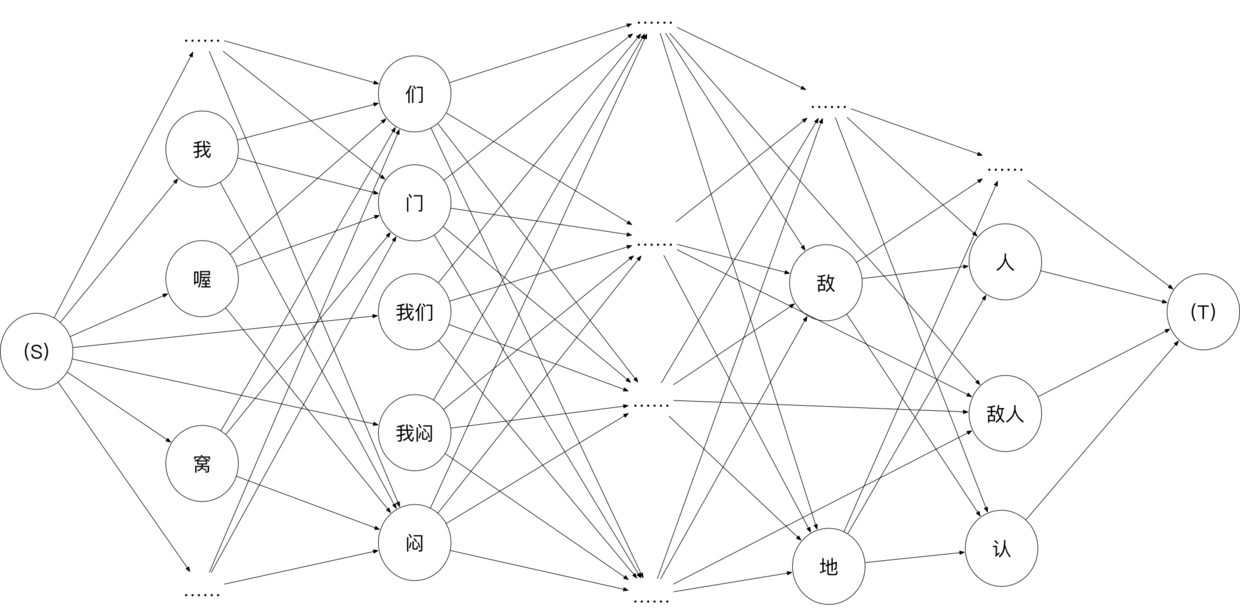public class SLMGraph : Graph
{
public SLMGraph(LexiconGraph lexicon_graph)
{
/**
* 顶点的数量比词组的总数多两个，分别用作起始顶点和终止顶点
*/
this.vertex_count = lexicon_graph.EdgeCount + 2;
InitializeVertex();
for (int i = 0; i < vertex_count; i++)
{
vertex[i].data = new VertexData();
}

//设置所有的顶点的词组信息
foreach (Edge e in lexicon_graph.Edges)
{
int id = (e.data as LexiconGraph.EdgeData).id;
(vertex[id].data as VertexData).phrase = (e.data as LexiconGraph.EdgeData).phrase;
}

(vertex.data as VertexData).phrase = "(S)";
(vertex[vertex_count - 1].data as VertexData).phrase = "(T)";

//创建从起始顶点指出的边
foreach (Edge e in lexicon_graph.Vertexes.to)
{
int id = (e.data as LexiconGraph.EdgeData).id;
}

//创建指向终止顶点的边
foreach (Edge e in lexicon_graph.Vertexes[lexicon_graph.VertexCount - 1].from)
{
int id = (e.data as LexiconGraph.EdgeData).id;
AddEdge(id, vertex_count - 1, new Weight().setValue(1));
}

//创建图中的其它边
for (int i = 0; i < lexicon_graph.VertexCount; i++)
{
foreach (Edge eprev in lexicon_graph.Vertexes[i].from)
{
int prev_id = (eprev.data as LexiconGraph.EdgeData).id;
string prev_phrase = (eprev.data as LexiconGraph.EdgeData).phrase;

foreach (Edge esucc in lexicon_graph.Vertexes[i].to)
{
int succ_id = (esucc.data as LexiconGraph.EdgeData).id;
string succ_phrase = (esucc.data as LexiconGraph.EdgeData).phrase;
}
}
}
//处理所有的未知的转移概率（权重）
ProbabilityLib.Instance.dealUnknownWeights();

}

/**
* 获得二元转移概率
*/
Weight GetBigram(string prev_phrase, string succ_phrase)
{
return ProbabilityLib.Instance.GetBigram(prev_phrase, succ_phrase);
}
/**
* 获得一元转移概率
*/
Weight GetUnigram(string phrase)
{
return ProbabilityLib.Instance.GetUnigram(phrase);
}

protected Edge AddEdge(int from, int to, Weight weight)
{
e.data = new EdgeData();
(e.data as EdgeData).weight = weight;
return e;
}

/**
*
* 用于携带转移概率的边数据类
*/
public class EdgeData
{
public Weight weight = null;
}

/**
* 用于携带词组的顶点数据类
*/
private class VertexData
{
public string phrase = null;

public override string ToString()
{
return phrase;
}
}
}


### 查询转移概率

    public class Weight
{
const double infinitesimal = 1e-100;
double Cinfinitesimal = CalculateWeight(infinitesimal);
/**
* 保存统计概率
*/
public double trueWeight = 0;
/**
* 保存取自然对数后的转移概率
*/
public double calculateWeight = 0;
/**
* 以下两个对象是后备的转移概率；
* 假使不存在关于“我 们”这一2元组的统计概率，则尝试取“我 <unknown>”的统计概率，
* 若无，则再尝试取“<unknown> 们”的统计概率，若还无，则返回1e-100；
*/
public Weight nextWeight1;
public Weight nextWeight2;
/**
* 标记该对象有无被设置过
*/
bool isSet = false;

public double TrueWeight
{
get
{
if (!isSet)
{
if (nextWeight1 != null)
{
return nextWeight1.TrueWeight;
}
else if (nextWeight2 != null)
{
return nextWeight2.TrueWeight;
}
return infinitesimal;
}
return trueWeight;
}
}

public Weight()
{

}

public virtual double value()
{
if (!isSet)
{
if (nextWeight1 != null)
{
return nextWeight1.value();
}
else if (nextWeight2 != null)
{
return nextWeight2.value();
}
return Cinfinitesimal;
}

return calculateWeight;
}

public Weight setValue(double weight)
{
isSet = true;

nextWeight1 = null;//该对象有值，无必要保持后备
nextWeight2 = null;
this.trueWeight = weight;
calculateWeight = CalculateWeight(weight);
return this;
}

protected static double CalculateWeight(double weight)
{
return Math.Log(weight);
}
}


    public class BiWeight : Weight
{

Weight delta;
/**
* 避免重复计算
*/
Boolean isDone = false;

public BiWeight(Weight w1, Weight w2, Weight delta) : base()
{
this.nextWeight1 = w1;
this.nextWeight2 = w2;
this.delta = delta;
}
public override double value()
{
if (!isDone)
{
trueWeight = nextWeight1.TrueWeight * nextWeight2.TrueWeight * (Math.E + delta.TrueWeight);
calculateWeight = CalculateWeight(trueWeight);
}
return calculateWeight;
}

}


        const double infinitesimal = 1e-100;
const string unknown = "<unknown>";
Dictionary<long, Weight> weights = new Dictionary<long, Weight>();
Dictionary<long, Weight> unknownWeights = new Dictionary<long, Weight>();



weights对象是用于保存已查询的转移概率的字典，其道理也是基于用户输入的渐进性特点。其键的类型为long，我们将使用字符串的哈希值作为键值；

unknownWeights对象是用于保存未知的待查询的转移概率的字典。

    internal Weight GetUnigram(string phrase)
{
long key = hashCode(phrase);
if (!weights.ContainsKey(key))
{
if (!unknownWeights.ContainsKey(key))
{
var w = new Weight();
return w;
}
else
{
return unknownWeights[key];
}
}
else
{
return weights[key];
}
}


    internal Weight GetBigram(string prev_phrase, string succ_phrase)
{
long key = hashCode(prev_phrase + " " + succ_phrase);
Weight delta;
List<Weight> list = new List<Weight>();
if (!weights.ContainsKey(key))
{
if (!unknownWeights.ContainsKey(key))
{
delta = new Weight();
long key2 = hashCode(prev_phrase + " " + unknown);//添加后备转移概率1
if (!weights.ContainsKey(key2))
{
if (!unknownWeights.ContainsKey(key2))
{
var w2 = new Weight();
delta.nextWeight1 = w2;
}
else
{
delta.nextWeight1 = unknownWeights[key2];
}
}
else
{
delta.nextWeight1 = weights[key2];
}
long key3 = hashCode(unknown + " " + succ_phrase);//添加后备转移概率2
if (!weights.ContainsKey(key3))
{
if (!unknownWeights.ContainsKey(key3))
{
var w3 = new Weight();
delta.nextWeight2 = w3;
}
else
{
delta.nextWeight2 = unknownWeights[key3];
}

}
else
{
delta.nextWeight2 = weights[key3];
}

}
else
{
delta = unknownWeights[key];
}

}
else
{
delta = weights[key];
}
return new BiWeight(GetUnigram(prev_phrase), GetUnigram(succ_phrase), delta);
}


    public void dealUnknownWeights()
{
var array = unknownWeights.ToArray();
StringBuilder s = new StringBuilder("select * from hashtable where ");
bool done = false;
for (int i = 0; i < array.Length;)
{
if (done)
{
s.Append(" or ");
}
done = true;
s.Append("hashcode = " + array[i++].Key);
if (i % 998 == 0)//一次查询不能超过1000个，只能分段查询；
{
s.Append(";");
done = false;
getProbability(s);
s = new StringBuilder("select * from hashtable where ");
}
}

if (array.Length % 998 != 0)
{
s.Append(";");
getProbability(s);
}
//最后将经过处理的Weight对象都放入weights中，表示其值已知，无论有没有被查询到；
foreach (var i in unknownWeights)
{
}
unknownWeights.Clear();
}

void getProbability(StringBuilder s)
{
var list = DataBase.Query<HashTable>(s.ToString());

foreach (var v in list)
{
var w = unknownWeights[v.HashCode];
w.setValue(v.Probability);
}
}


### 求最短路径

public class DepthFirstOrder {
bool[] marked;
Stack<int> reversePost;
public DepthFirstOrder(Graph G) {
reversePost = new Stack<int>();
marked = new bool[G.Vertexes.Length];
for (int i = 0; i < G.Vertexes.Length; i++) {
if (!marked[i]) dfs(G, i);
}
}

public Stack<int> ReversePost { get { return reversePost; } }

private void dfs(Graph g, int v) {
marked[v] = true;
foreach (var i in g.adj(v)) {
if (!marked[i]) dfs(g, i);
}
reversePost.Push(v);
}
}


    public class AcyclicSP
{
Graph.Edge[] edgeTo;
double[] distTo;
public AcyclicSP(Graph g, int s)
{
edgeTo = new Graph.Edge[g.Vertexes.Length];
distTo = new double[g.Vertexes.Length];
for (int v = 0; v < g.Vertexes.Length; v++)
{
distTo[v] = double.PositiveInfinity;
}
distTo[s] = 0;

DepthFirstOrder order = new DepthFirstOrder(g);//拓扑排序
foreach (var v in order.ReversePost)
{
relax(g, v);
}

}
/**
* 这就是所谓的放松操作
*/
private void relax(Graph g, int v)
{
{
int w = g.getIndex(e.to);
var v2 = (e.data as SLMGraph.EdgeData).weight.value();
double v1 = distTo[v] - v2;//此处使用负号，是对求自然对数后的转移概率取反，使其变成正数；
if (distTo[w] > v1)
{
distTo[w] = v1;
edgeTo[w] = e;
}
}
}
/**
* 输出最短路径上的顶点
*/
public Stack<Graph.Edge> pathTo(Graph g, int v)
{
Stack<Graph.Edge> path = new Stack<Graph.Edge>();
bool done = false;
for (var e = edgeTo[v]; e != null; e = edgeTo[g.getIndex(e.from)])
{
if (done) path.Push(e);
done = true;
}
return path;
}
}


### 模型整合与实验

public class SuiHan2GramModel {

private string sentence;

public SuiHan2GramModel(string sheng, string yun) {
LexiconGraph graph = new LexiconGraph(sheng, yun);
SLMGraph sgraph = new SLMGraph(graph);
AcyclicSP sp = new AcyclicSP(sgraph, 0);
var path = sp.pathTo(sgraph, sgraph.VertexCount - 1);
StringBuilder s = new StringBuilder();
foreach (var i in path) {
s.Append(i.to.ToString());
}
sentence = s.ToString();
}

public string Sentence {
get {
return sentence;
}
}
}


static void makeSentence(string sheng, string yun)
{
Stopwatch s = new Stopwatch();
s.Start();
var gram = new SuiHan2GramModel(sheng, yun);
s.Stop();

Console.WriteLine(gram.Sentence + s.ElapsedMilliseconds);
}

public static void Main(string[] args)
{
//在此之前应当执行初始数据库的操作

}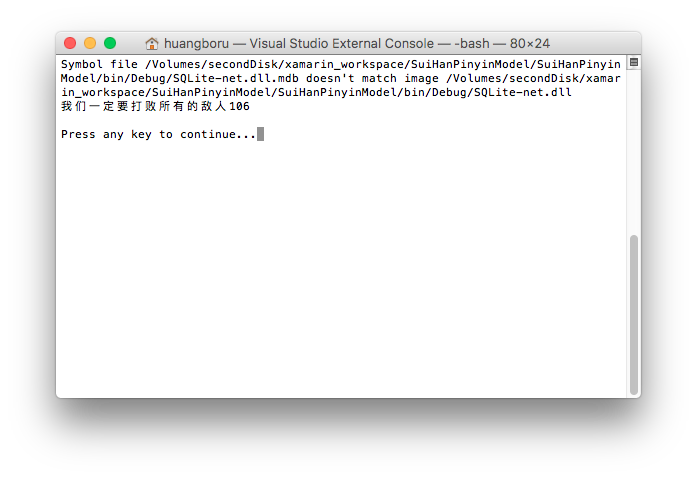106ms的运行时间还是有点长的，但是请注意，这里我们并没有引入用户的输入渐进性，而是直接对整个拼音序列执行整句，所以我们应当模仿用户实际输入的渐进过程再做次实验。

    public static void Main(string[] args)
{
const string sheng = "wmydydbsyddr";
for (int i = 1; i <= sheng.Length; i++)
{
makeSentence(sheng.Substring(0, i), yun.Substring(0, i));
}
}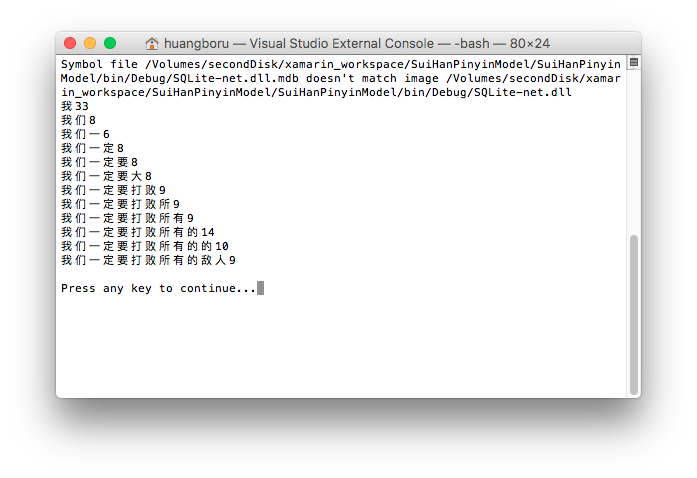9ms！应该说是非常不错的成绩，用时最长的反而是查询最短的时候，说明此时初始化的开销成为了整句过程的大头，也充分说明了对词组查询和概率查询的缓存的重要性。一个10ms级的整句引擎理论上是不会导致用户在输入时出现卡滞现象的，已经可以满足实用需要了。

### 要点回顾

• 统计数据的哈希化
• 建立unique索引
• 对取词进行排序并限制取词的数量
• 对取词进行缓存，以利用实际输入过程的渐进性
• 对概率查询进行合并
• 对概率查询进行缓存，以利用实际输入过程的渐进性
• 使用拓扑排序求解最短路径

### 写在最后